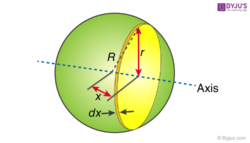# Moment of Inertia of Solid Sphere

Inertia is the property of the matter by which it resists any change in the state of rest or state of motion. The larger the inertia of the body, the greater is the force required to make changes in the velocity in the given time interval. Similarly, the moment of inertia is that property of the object to resist any change in the state of rotatory motion. The larger the moment of inertia the larger is the amount of torque required to cause any change in the angular velocity. The torque is the force that causes the object to rotate about an axis.

## How to Find Moment of Inertia of Solid SphereA uniform solid sphere of mass M and radius R is taken. Moment of inertia is calculated about an axis passing through its centre. The solid sphere should be sliced to infinitesimally thin solid cylinders. The moment of inertia of a solid cylinder is given as

I = (½)MR2

For infinitesimally small cylinder moment of inertia can be written as

dI = (½)r2dm

dm = ρ dV,

where ρ is the density of the solid cylinder and dV is the volume.

dV = πr2dx

Putting the value of dV in dm

dm = ρπr2dx

Substituting dm into dI

dI = (½)ρπrdx

In the above image r, x and R form a triangle. Hence, by using Pythagoras theorem we have

r2 = R2 – x2

Substituting in the equation for dI

dI = (½)ρπ(R2 – x2)dx

Integrating between -R and R

$I = \frac{1}{2}\rho \pi \int_{-R}^{R}(R^{2}-x^{2})dx$

Simplifying the above equation we get

I = (½)ρπ(16/15)R5

The density of the sphere, ρ = M/V

ρ = M/[(4/3)πR3]

Substituting ρ in the equation of I, we will have

I = ((½) x M/[(4/3)πR3] x π(16/15)R5

I = (⅔)MR2

Moment of inertia of solid sphere, I = (⅔)MR2